### Basic Definitions and Notation

The gradient of a function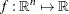is the vector of all the first partial derivatives of f and is denoted by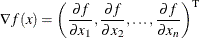where the superscript T denotes the transpose of a vector.

The Hessian matrix of f, denoted by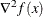, or simply by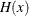, is ansymmetric matrix whose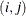element is the second partial derivative of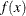with respect toand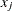. That is,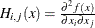.

Consider the vector function,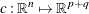, whose first p elements are the equality constraint functions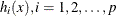, and whose last q elements are the inequality constraint functions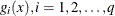. That is,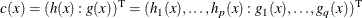The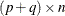matrix whose ith row is the gradient of the ith element of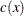is called the Jacobian matrix of(or simply the Jacobian of the NLP problem) and is denoted by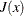. You can also use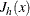to denote the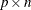Jacobian matrix of the equality constraints and use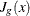to denote the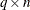Jacobian matrix of the inequality constraints. It is easy to see that